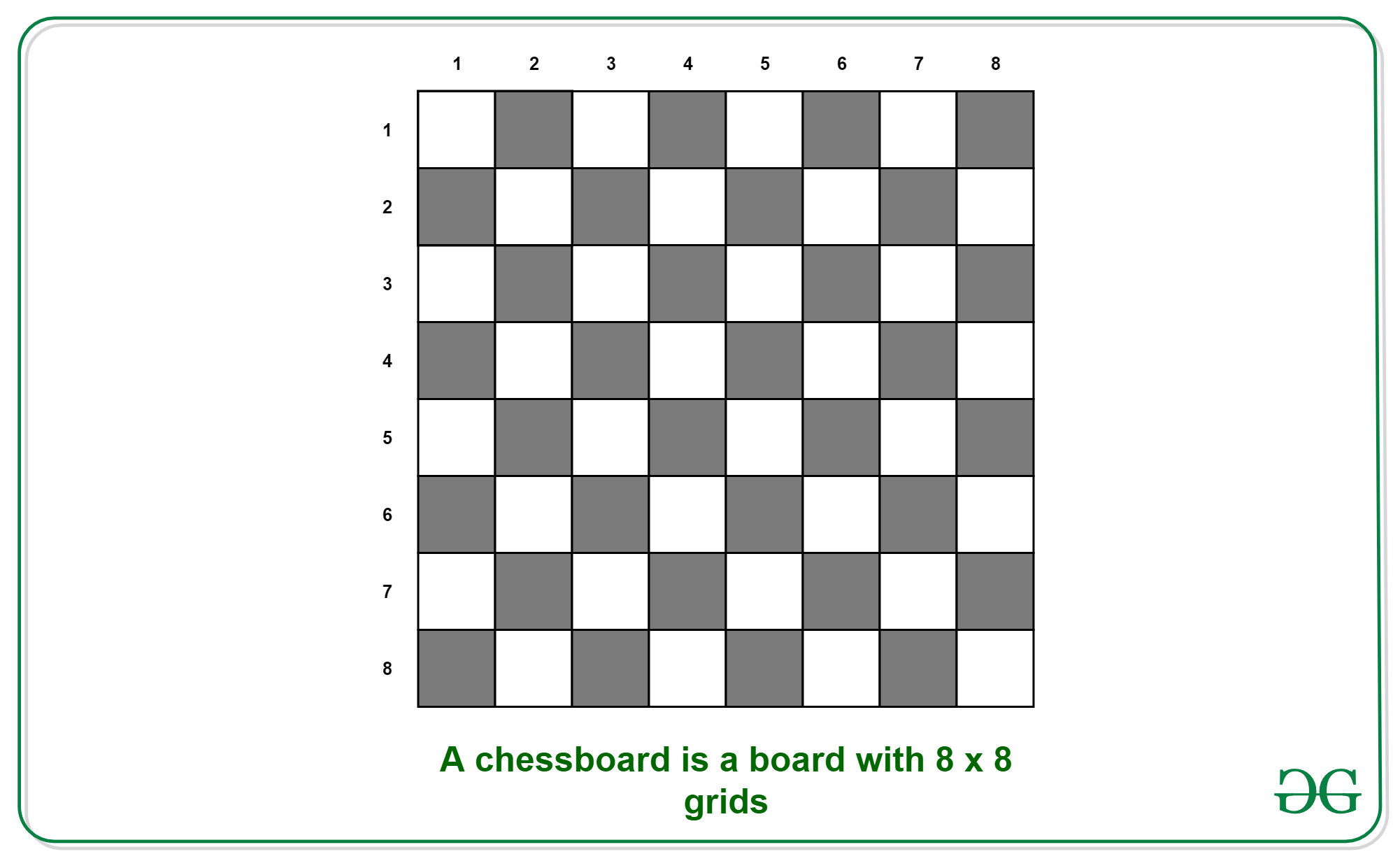Open In App

# Puzzle | Program to find number of squares in a chessboard

Puzzle: You are provided with a chessboard and are asked to find the number of squares in it. A chessboard is a board with 8 x 8 grids in it as represented below.Solution: Looking closely at the chessboard we can see that in addition to the 1 x 1 square, there can be a combination of 2 x 2, 3 x 3, 4 x 4, 5 x 5, 6 x 6, 7 x 7, and 8 x 8 squares too. To get the total number of squares we need to find all the squares formed.

```1 x 1: 8 * 8 = 64 squares.
2 x 2: 7 * 7 = 49 squares.
3 x 3: 6 * 6 = 36 squares.
4 x 4: 5 * 5 = 25 squares.
5 x 5: 4 * 4 = 16 squares.
6 x 6: 3 * 3 = 9 squares.
7 x 7: 2 * 2 = 4 squares.
8 x 8: 1 * 1 = 1 square.```

Therefore, we have in all = 64 + 49 + 36 + 25 + 16 + 9 + 4 + 1 = 204 squares in a chessboard.

General Process
Given an n x n grid, count squares in it.

Examples:

```Input: n = 2
Output: 5 (4 squares of 1 unit + 1 square of 2 units)

Input: n = 3
Output: 14 (9 squares of 1 unit + 4 square of 2 units
+ 1 square of 1 unit) ```

For a grid of size n*n the total number of squares formed are:

`1^2 + 2^2 + 3^2 + ... + n^2 = n(n+1)(2n+1) / 6 `

Below is the implementation of the above formula. Since the value of n*(n+1)*(2n+1) can cause overflow for large values of n, below are some interesting tricks used in the program.

1. long int is used in return.
2. n * (n + 1) / 2 is evaluated first as the value n*(n+1) will always be a multiple of 2.

Note that overflow may still happen, but the above tricks just reduce the chances of an overflow.

## C++

 `// C++  find number of squares in a``// chessboard``#include ``using` `namespace` `std;` `// Function to return count of squares;``long` `long` `int` `countSquares(``int` `n)``{``  ``return` `(n * (n + 1) / 2) * (2 * n + 1) / 3;``}` `// Driver Code``int` `main()``{` `  ``int` `n = 4;``  ``cout << countSquares(n);``  ``return` `0;``}` `//vermay87    ``

## Java

 `// Java  find number of squares in a``// chessboard`  `class` `GFG``{``    ``// Function to return count of squares;``    ``static` `int` `countSquares(``int` `n)``    ``{``        ``// A better way to write n*(n+1)*(2n+1)/6``        ``return` `(n * (n + ``1``) / ``2``) * (``2` `* n + ``1``) / ``3``;``    ``}``    ` `    ``// Driver code``    ``public` `static` `void` `main (String[] args)``    ``{``        ``int` `n = ``3``;``        ``System.out.println(``"Count of squares is "``                           ``+countSquares(n));``    ``}``}` `// This code is contributed by Anant Agarwal.`

## Python3

 `# python code to find number``# of squares in a chessboard` `# Function to return count``# of squares;``def` `countSquares(n):``    ` `    ``# better way to write``    ``# n*(n+1)*(2n+1)/6``    ``return` `((n ``*` `(n ``+` `1``) ``/` `2``)``           ``*` `(``2` `*` `n ``+` `1``) ``/` `3``)``    ` `# Driver code``n ``=` `4``print``(``"Count of squares is "``,``              ``countSquares(n))` `# This code is contributed by sam007.`

## C#

 `// C# find number of squares in a``// chessboard``using` `System;` `public` `class` `GFG {``    ` `    ``static` `int` `countSquares(``int` `n)``    ``{``        ``// A better way to write``        ``// n*(n+1)*(2n+1)/6``        ``return` `(n * (n + 1) / 2)``                ``* (2 * n + 1) / 3;``    ``}``    ` `    ``// Driver code``    ``public` `static` `void` `Main ()``    ``{``        ``int` `n = 4;``        ``Console.WriteLine(``"Count of"``                     ``+ ``"squares is "``                 ``+ countSquares(n));``    ``}``}` `// This code is contributed by Sam007.`

## PHP

 ``

## Javascript

 ``

Output:

`Count of squares is 30`

Time Complexity: O(1)

Auxiliary Space: O(1)